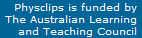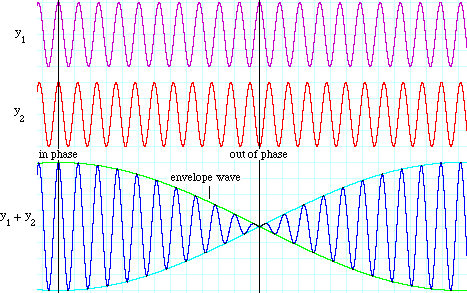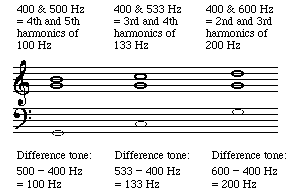# Interference beats and Tartini tones

 Beats are often observed between two vibrations with similar frequencies. The beat frequency equals the difference between frequencies of the beating signals. When sound signals interfere, the beat signal can sometimes be heard as a separate note: the Tartini tone. Both are useful and important in practice for measuring frequencies and for tuning musical instruments. (It is also worth looking at for the insight it gives to Heisenberg's Uncertainty Principle, as we shall see below.) This background page to the multimedia chapter Interference gives sound files and derivations. Introduction Explanation and derivation Sample sound files (beats and Tartini tones for a range of pure sine waves) Tartini tones Varying the beat frequency (with video clips) Interference and consonance (with video clips) Using beats and harmonics to tune a guitar What it has to do with Heisenberg's Uncertainty Principle (separate page)### Introduction

Let's consider two waves with the same amplitude A, and frequencies f1 and f2 that are not very different. Before we do any maths, we can see what will happen by looking at the diagram. In this plot, the wave depicted by the red graph plus that depicted by the purple one gives that represented by the blue curve. The horizontal axis is usually time. Suppose that the waves start out in phase, so that they add up, as shown at the left of the diagram. The wave depicted by the red graph has a slightly higher frequency than that depicted by the purple one, so it gradually gains on it, and eventually gets one half cycle out of phase. At this point, the two component waves cancel out, and the amplitude of the blue curve is near zero. After an equal interval of time, they get back in step again, as shown.

If the waves are sound waves, what will this sound like? Well, provided that the difference in frequency is small enough, the resultant wave will sound loud when the two components are in phase and soft or absent when they are out of phase. The frequency of the blue wave is, if you look carefully, about halfway between that of the red and the purple. So we should hear a wave of intermediate frequency, getting regularly louder and softer. This is the acoustical example of the phenomenon of interference beats.

(If the difference in frequencies is greater than a few tens of cycles per second, we won't recognise the very short period of cancellation as being soft, and in fact we'll get some interesting effects, to which we return later.)

### Explanation and derivationNow let's get quantitative. For the purple and red waves respectively, let's write
y1 = A cos (2π f1)t     and y2 = A cos (2π f2t),     so

ytotal = y1 + y2 = A {cos (2π f1t) + cos (2π f2t)}    (1).

To get any further, we need the trigonometric identity that
cos (a+b) = cos a * cos b - sin a * sin b,     from which it follows that
cos (a-b) = cos a * cos b + sin a * sin b.
Adding these two equations gives us
cos (a+b) + cos (a-b) = 2 cos a * cos b    (2).
We now use this identity by making the substitions
a = 2π (f1t + f2t)/2   and   b = 2π (f1t - f2t)/2,     so
a + b = 2π f1t       and       a - b = 2π f2t.
We now substitute this into equation (2) to get
cos (2π f1t) + cos (2π f2t) = 2 cos (2π (f1t + f2t)/2) * cos (2π (f1t - f2t)/2)     (3)
Now we recognise (f1 + f2)/2 as the average frequency fav and (f1 - f2) as the frequency difference Δf.
Finally, we multiply (3) by A to get:
ytotal = y1 + y2 = 2A {cos (2π Δf/2)t} * {cos (2π fav)t}     (4).

On the diagram below, we see that the maximum amplitude of the compound wave is twice that of the two interfering waves. The carrier wave indeed has the average frequency, as you can verify by counting cycles on the diagram.### Tartini Tones

 If you have listened to the sound samples above at a reasonably high sound level, you may have heard Tartini tones or difference tones, particularly on numbers 10, 11 and 12. Tartini tones sound like a low pitched buzzing note with a frequency equal to the difference between the frequencies of the two interfering tones. If you play the sound files number 10, 11 and 12 moderately loudly, you will hear Tartini tones with frequencies 100, 133 and 200 Hz, corresponding approximately to the notes G2, C3, G3, as shown in the illustration at right. (See note names to convert among notes, notations and frequencies.) There is more about this on the FAQ in music acoustics. As well as the sound samples above, there are some more samples showing Tartini tones below. We also have a page on Tartini tones and their relation to temperament, which has more examples.Text

### Varying the beat frequency

Here are some film clips made with oscilloscopes to illustrate the beats and Tartini tones. The laboratory set-up is described here. The first shows signals that differ in frequency by 1 Hz.

Next, we increase the higher frequency, so that the difference increases smoothly from 1 to 10 Hz. How does this sound to you?

Most people agree that the sound in the clip above is a single pure tone, with amplitude varying as beats, from slow to fast. (By the way, the window on the spectrum analyser (screen bottom right) is not long enough to resolve the two frequencies.)

In the clip below, the lower frequency is still 400 Hz, but the higher is increased smoothly from 410 to 435 Hz. What do you hear?

You may have heard rapid beats initially, increasing at first. What after that? For some listeners, the end of the clip is distinctly two different notes. (The spectrum analyser also resolves them.) For others, it just sounds like an unclear sound. Some people refer to a range lying between fast beats and separate notes as a region of roughness. You can investigate this further by listening again, by using the sample sound files above, or by downloading the two channel sine wave generator from our lab site (Mac only at this stage).

### Interference and consonance

The ratios 3:2 and 5:4 are called (by many Western people, at least), musical consonances (in just intonation). In this example, one tone remains constant at 400 Hz. The other is varied rapidly from 400 to 500 Hz, where it pauses briefly, before increasing to 600 Hz. You may hear a descending Tartini tone during the first of the variations. If you do not hear it at first, you might try increasing the volume and using headphones. Do you hear a Tartini tone during the second?

Here are some more consonances in just intonation.

and here a scale constructed using the notes used the consonances given above, plus two consonances at 5:4 and 3:2 based on the fifth note in the scale.

However, this has taken us some distance from beats and Tartini tones. For more detail and explanation, go to our page on Tartini tones and their relation to temperament.

### Tuning a guitar with beats and harmonicsHere I use the fourth 'harmonic' on the low E string and the third 'harmonic' on the A string. The E string plays the note E2 so its fourth harmonic is E4, two octaves above. The A string plays A2, so its third harmonic is also E4, a (just) twelfth above, as shown in the diagram at right. In this video clip, I have already tuned the E string, so here I adjust the tuning of the A string so its third 'harmonic' stops beating with the fourth 'harmonic' of the E string.

Look at the soundtrack. From 0.6 to 1.0 s, only the E string sounds, so there are no beats. From 1.0 to 2.4 s, there are beats at about 8 Hz, so the third 'harmonic' of the A string is about 8 Hz flat, so the A string is 8/3 Hz ~ 3 Hz flat. From 2.5 to about 5 s, as I increase the tension in the A string, we see and hear that the beat frequency decreases. Listening and looking closely after about 5 s, we can still hear beats, but their frequency is less than half a Hz. This can be reduced a little in a second tuning step, not shown here. (Using this method to tune all strings, and its limitations, are discussed on Strings and harmonics.)

In the clip above, we notice that, because of the finite ring time of the strings, there is a limit to the precision of the tuning. Which is a good time to introduceThis work is licensed under a Creative Commons License.